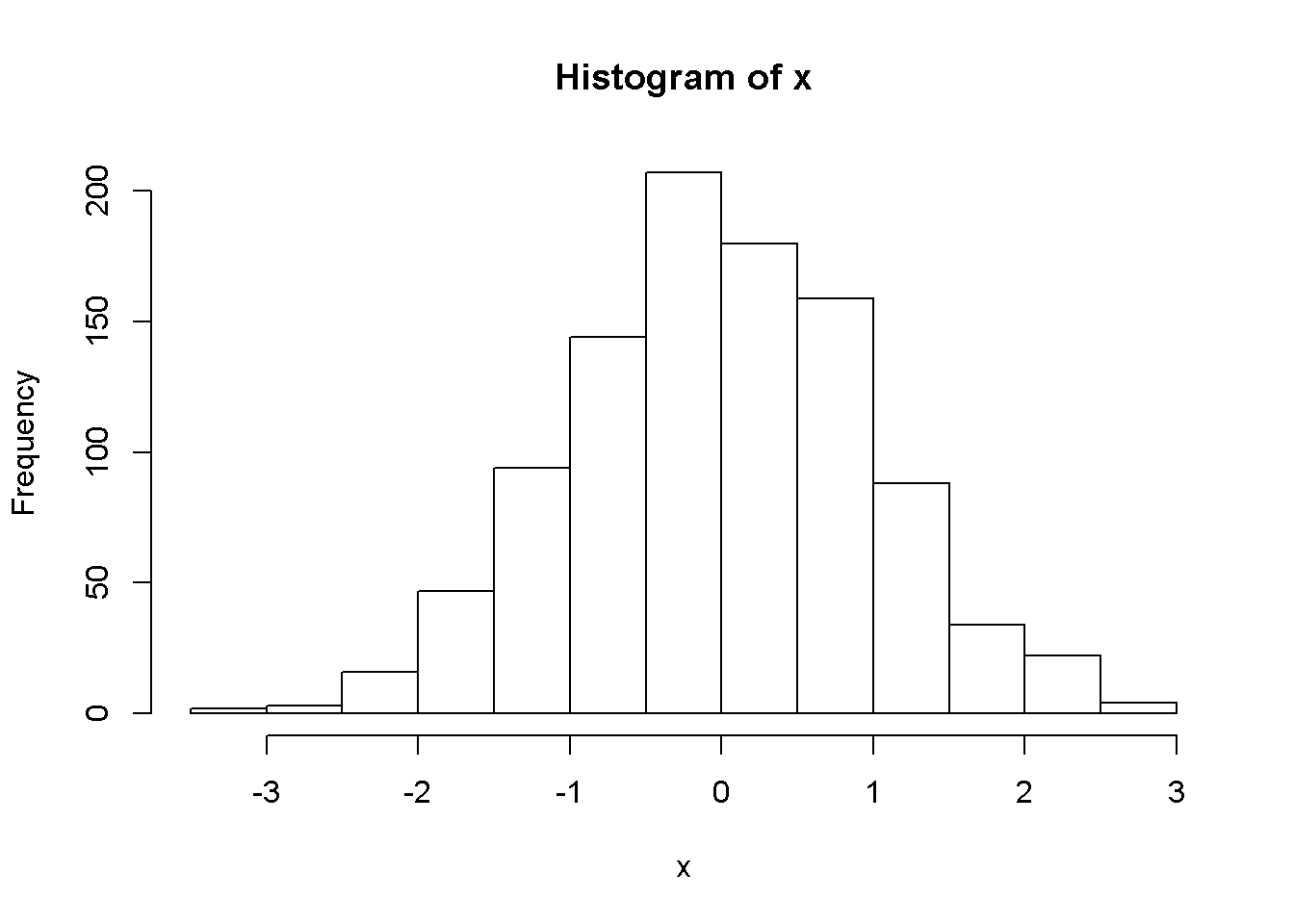# The BrailleR package

## Introduction

This vignette briefly demonstrates the functions of the BrailleR package. It is far from complete as it is currently a work in progress file for testing various aspects of vignette creation using the knitr package.

## Histograms

The first and most commonly used example of the value of the BrailleR package to a blind user is the creation of a histogram.

x=rnorm(1000)
VI(hist(x))## This is a histogram, with the title: Histogram of x
##  x is marked on the x-axis.
## There are a total of 1000 elements for this variable.
## It has 13 bins with equal widths, starting at -3.5 and ending at 3 .
## The mids and counts for the bins are:
## mid = -3.25  count = 2
## mid = -2.75  count = 3
## mid = -2.25  count = 16
## mid = -1.75  count = 47
## mid = -1.25  count = 94
## mid = -0.75  count = 144
## mid = -0.25  count = 207
## mid = 0.25  count = 180
## mid = 0.75  count = 159
## mid = 1.25  count = 88
## mid = 1.75  count = 34
## mid = 2.25  count = 22
## mid = 2.75  count = 4

The mean $$\mu$$ of this random sample is -0.0192 which of course should be near enough to zero.